# Lab 6.1 CASE Statements

 Team-Fly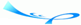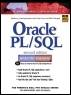Oracle® PL/SQL® Interactive Workbook, Second EditionBy Benjamin Rosenzweig, Elena Silvestrova Table of Contents Chapter 6.  Conditional Control: Case Statements

Lab Objectives

After this Lab, you will be able to:

• Use CASE Statements

• Use Searched CASE Statements

A CASE statement has two forms: CASE and searched CASE. A CASE statement allows you to specify a selector that determines which group of actions to take. A searched CASE statement does not have a selector; it has search conditions that are evaluated in order to determine which group of actions to take.

#### CASE Statements

A CASE statement has the following structure:

` CASE SELECTOR     WHEN EXPRESSION 1 THEN STATEMENT 1;     WHEN EXPRESSION 2 THEN STATEMENT 2;     …     WHEN EXPRESSION N THEN STATEMENT N;     ELSE STATEMENT N+1;  END CASE; `

The reserved word CASE marks the beginning of the CASE statement. A selector is a value that determines which WHEN clause should be executed. Each WHEN clause contains an EXPRESSION and one or more executable statements associated with it. The ELSE clause is optional and works similar to the ELSE clause used in that IF-THEN-ELSE statement. END CASE is a reserved phrase that indicates the end of the CASE statement. This flow of the logic from the preceding structure of the CASE statement is illustrated in Figure 6.1.

##### Figure 6.1. CASE Statement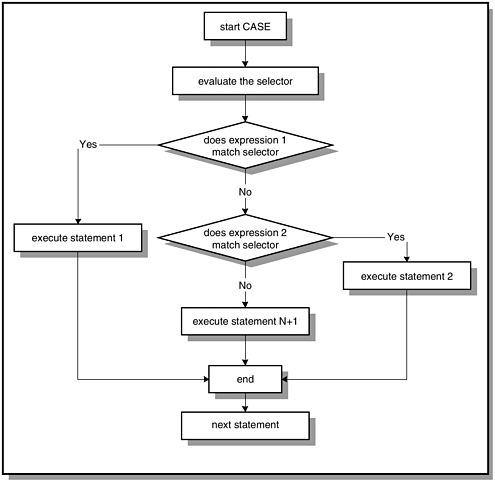Note that the selector is evaluated only once. The WHEN clauses are evaluated sequentially. The value of an expression is compared to the value of the selector. If they are equal, the statement associated with a particular WHEN clause is executed, and subsequent WHEN clauses are not evaluated. If no expression matches the value of the selector, the ELSE clause is executed.

Recall the example of the IF-THEN-ELSE statement used in the previous chapter.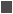FOR EXAMPLE

` DECLARE     v_num NUMBER := &sv_user_num;  BEGIN     -- test if the number provided by the user is even     IF MOD(v_num,2) = 0  THEN        DBMS_OUTPUT.PUT_LINE (v_num||' is even number');     ELSE        DBMS_OUTPUT.PUT_LINE (v_num||' is odd number');     END IF;     DBMS_OUTPUT.PUT_LINE ('Done');  END; `

Consider the new version of the same example with the CASE statement instead of the IF-THEN-ELSE statement.FOR EXAMPLE

` DECLARE     v_num NUMBER := &sv_user_num;     v_num_flag NUMBER;  BEGIN     v_num_flag := MOD(v_num,2);         -- test if the number provided by the user is even     CASE v_num_flag        WHEN 0 THEN           DBMS_OUTPUT.PUT_LINE (v_num||' is even number');        ELSE           DBMS_OUTPUT.PUT_LINE (v_num||' is odd number');     END CASE;     DBMS_OUTPUT.PUT_LINE ('Done');  END; `

In this example, a new variable, v_num_flag, is used as a selector for the CASE statement. If the MOD function returns 0, then the number is even; otherwise it is odd. If v_num is assigned the value of 7, this example produces the following output:

` Enter value for sv_user_num: 7  old   2:    v_num NUMBER := &sv_user_num;  new   2:    v_num NUMBER := 7;  7 is odd number  Done  PL/SQL procedure successfully completed. `

#### Searched CASE Statements

A searched CASE statement has search conditions that yield Boolean values: TRUE, FALSE, or NULL. When a particular search condition evaluates to TRUE, the group of statements associated with this condition is executed. This is indicated as follows:

` CASE     WHEN SEARCH CONDITION 1 THEN STATEMENT 1;     WHEN SEARCH CONDITION 2 THEN STATEMENT 2;     …     WHEN SEARCH CONDITION N THEN STATEMENT N;     ELSE STATEMENT N+1;  END CASE; `

When a search condition evaluates to TRUE, control is passed to the statement associated with it. If no search condition yields TRUE, then statements associated with the ELSE clause are executed. This flow of logic from the preceding structure of the searched CASE statement is illustrated in Figure 6.2.

##### Figure 6.2. Searched CASE Statement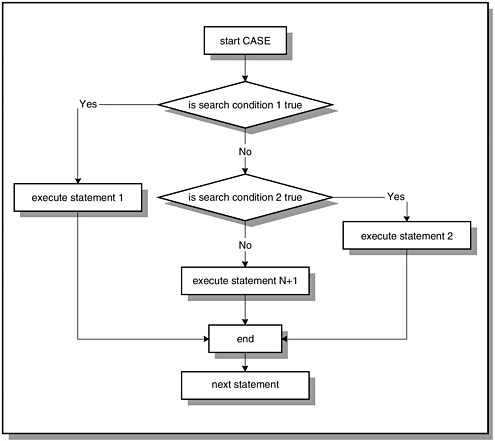Consider the modified version of the example that you have seen previously in this lab.FOR EXAMPLE

` DECLARE     v_num NUMBER := &sv_user_num;  BEGIN     -- test if the number provided by the user is even     CASE        WHEN MOD(v_num,2) = 0 THEN           DBMS_OUTPUT.PUT_LINE (v_num||' is even number');        ELSE           DBMS_OUTPUT.PUT_LINE (v_num||' is odd number');     END CASE;     DBMS_OUTPUT.PUT_LINE ('Done');  END; `

Notice that this example is almost identical to the previous example.

In the previous example, the variable v_num_flag was used as a selector, and the result of the MOD function was assigned to it. The value of the selector was then compared to the value of the expression. In this example, you are using a searched CASE statement, so there is no selector present. The variable v_num is used as part of the search conditions, so there is no need to declare variable v_num_flag. This example produces the same output when the same value is provided for the v_num:

` Enter value for sv_user_num: 7  old   2:    v_num NUMBER := &sv_user_num;  new   2:    v_num NUMBER := 7;  7 is odd number  Done  PL/SQL procedure successfully completed. `

#### Differences Between CASE and Searched CASE Statements

It is important to note the differences between the CASE and searched CASE statements. You have seen that the searched CASE statement does not have a selector. In addition, its WHEN clauses contain search conditions that yield a Boolean value similar to the IF statement, not expressions that can yield a value of any type except a PL/SQL record, an index-by-table, a nested table, a vararray, BLOB, BFILE, or an object type. You will encounter some of these types in the future chapters. Consider the following two code fragments based on the examples you have seen earlier in this chapter.FOR EXAMPLE

` DECLARE     v_num NUMBER := &sv_user_num;     v_num_flag NUMBER;  BEGIN     v_num_flag := MOD(v_num,2);     -- test if the number provided by the user is even     CASE v_num_flag        WHEN 0 THEN           DBMS_OUTPUT.PUT_LINE (v_num||' is even number');  … `FOR EXAMPLE

` DECLARE     v_num NUMBER := &sv_user_num;  BEGIN     -- test if the number provided by the user is even     CASE        WHEN MOD(v_num,2) = 0 THEN  … `

In the first code fragment, v_num_flag is the selector. It is a PL/SQL variable that has been defined as NUMBER. Because the value of the expression is compared to the value of the selector, the expression must return a similar datatype. The expression '0' contains a number, so its datatype is also numeric. In the second code fragment, each searched expression evaluates to TRUE or FALSE just like conditions of an IF statement.

Next, consider an example of the CASE statement that generates a syntax error because the datatype returned by the expressions does not match the datatype assigned to the selector.FOR EXAMPLE

` DECLARE     v_num NUMBER := &sv_num;     v_num_flag NUMBER;  BEGIN     CASE v_num_flag        WHEN MOD(v_num,2) = 0 THEN           DBMS_OUTPUT.PUT_LINE (v_num||' is even number');        ELSE           DBMS_OUTPUT.PUT_LINE (v_num||' is odd number');     END CASE;     DBMS_OUTPUT.PUT_LINE ('Done');  END; `

In this example, the variable v_num_flag has been defined as a NUMBER. However, the result of each expression yields Boolean datatype. As a result, this example produces the following syntax error:

` Enter value for sv_num: 7  old   2:    v_num NUMBER := &sv_num;  new   2:    v_num NUMBER := 7;     CASE v_num_flag          *  ERROR at line 5:  ORA-06550: line 5, column 9:  PLS-00615: type mismatch found at 'V_NUM_FLAG' between  CASE operand and WHEN operands  ORA-06550: line 5, column 4:  PL/SQL: Statement ignored `

Consider a modified version of this example where v_num_flag has been defined as a Boolean variable.FOR EXAMPLE

` DECLARE     v_num NUMBER := &sv_num;     v_num_flag Boolean;  BEGIN     CASE v_num_flag        WHEN MOD(v_num,2) = 0 THEN           DBMS_OUTPUT.PUT_LINE (v_num||' is even number');        ELSE           DBMS_OUTPUT.PUT_LINE (v_num||' is odd number');     END CASE;     DBMS_OUTPUT.PUT_LINE ('Done');  END; `

If v_num is assigned the value of 7 again, this example produces the following output:

` Enter value for sv_num: 7  old   2:    v_num NUMBER := &sv_num;  new   2:    v_num NUMBER := 7;  7 is odd number  Done  PL/SQL procedure successfully completed. `

At first glance this seems to be the output that you would expect. However, consider the output produced by this example when the value of 4 is assigned to the variable v_num:

` Enter value for sv_num: 4  old   2:    v_num NUMBER := &sv_num;  new   2:    v_num NUMBER := 4;  4 is odd number  Done  PL/SQL procedure successfully completed. `

Notice that the second run of the example produced an incorrect output even though it did not generate any syntax errors. When the value 4 is assigned to the variable v_num, the expression

` MOD(v_num,2) = 0 `

yields TRUE, and it is compared to the selector v_num_flag. However, the v_num_flag has not been initialized to any value, so it is NULL. Because NULL does not equal to TRUE, the statement associated with the ELSE clause is executed.

#### Lab 6.1 Exercises

##### 6.1.1 Use the CASE Statement

In this exercise, you will use the CASE statement to display the name of a day on the screen based on the number of the day in a week. In other words, if the number of a day of the week is 3, then it is Tuesday.

Create the following PL/SQL script:

` -- ch06_1a.sql, version 1.0  SET SERVEROUTPUT ON  DECLARE     v_date DATE := TO_DATE('&sv_user_date', 'DD-MON-YYYY');     v_day VARCHAR2(1);  BEGIN     v_day := TO_CHAR(v_date, 'D');     CASE v_day        WHEN '1' THEN           DBMS_OUTPUT.PUT_LINE ('Today is Sunday');        WHEN '2' THEN           DBMS_OUTPUT.PUT_LINE ('Today is Monday');        WHEN '3' THEN           DBMS_OUTPUT.PUT_LINE ('Today is Tuesday');        WHEN '4' THEN           DBMS_OUTPUT.PUT_LINE ('Today is Wednesday');        WHEN '5' THEN           DBMS_OUTPUT.PUT_LINE ('Today is Thursday');        WHEN '6' THEN           DBMS_OUTPUT.PUT_LINE ('Today is Friday');        WHEN '7' THEN           DBMS_OUTPUT.PUT_LINE ('Today is Saturday');     END CASE;  END; `

Execute the script, and then answer the following questions:

 a) If the value of v_date equals '15-JAN-2002', what output is printed on the screen? b) How many times is the CASE selector v_day evaluated? c) Rewrite this script using the ELSE clause in the CASE statement. d) Rewrite this script using the searched CASE statement.

##### 6.1.2 Use the Searched CASE Statement

In this exercise, you will modify the script ch05_3d.sql used in the previous chapter. The original script uses the ELSIF statement to display a letter grade for a student registered for a specific section of course number 25. The new version will use a searched CASE statement to achieve the same result. Try to answer the questions before you run the script. Once you have answered the questions, run the script and check your answers. Note that you may need to change the values for the variables v_student_id and v_section_id as you see fit in order to test some of your answers.

Create the following PL/SQL script:

` -- ch06_2a.sql, version 1.0  SET SERVEROUTPUT ON  DECLARE     v_student_id NUMBER := 102;     v_section_id NUMBER := 89;     v_final_grade NUMBER;     v_letter_grade CHAR(1);  BEGIN     SELECT final_grade       INTO v_final_grade       FROM enrollment      WHERE student_id = v_student_id        AND section_id = v_section_id;     CASE        WHEN v_final_grade >= 90 THEN v_letter_grade := 'A';        WHEN v_final_grade >= 80 THEN v_letter_grade := 'B';        WHEN v_final_grade >= 70 THEN v_letter_grade := 'C';        WHEN v_final_grade >= 60 THEN v_letter_grade := 'D';        ELSE v_letter_grade := 'F';     END CASE;     -- control resumes here     DBMS_OUTPUT.PUT_LINE ('Letter grade is: '||        v_letter_grade);  END; `

Try to answer the following questions first, and then execute the script:

 a) What letter grade will be displayed on the screen: if the value of v_final_grade is equal to 60?if the value of v_final_grade is greater than 60 and less than 70?if the value of v_final_grade is NULL? b) How would you change this script so that a message "There is no final grade" is displayed if v_final_grade is null? In addition, make sure that the message "Letter grade is: " is not displayed on the screen. c) Rewrite this script, changing the order of the searched conditions as follows: ` CASE WHEN v_final_grade >= 60 THEN v_letter_grade := 'D'; WHEN v_final_grade >= 70 THEN v_letter_grade := 'C'; WHEN v_final_grade >= 80 THEN … WHEN v_final_grade >= 90 THEN … ELSE … `

Execute the script and explain the output produced.

This section gives you some suggested answers to the questions in Lab 6.1, with discussion related to how those answers resulted. The most important thing to realize is whether your answer works. You should figure out the implications of the answers here and what the effects are from any different answers you may come up with.

 a) If the value of v_date equals '15-JAN-2002', what output is printed on the screen? A1: Answer: Your output should look like the following:` Enter value for sv_user_date: 15-JAN-2002 old 2: v_date DATE := TO_DATE('&sv_user_date', 'DD- MON-YYYY'); new 2: v_date DATE := TO_DATE('15-JAN-2002', 'DD-MON- YYYY'); Today is Tuesday PL/SQL procedure successfully completed. `When the value of 15-JAN-2002 is entered for v_date, the number of the day of the week is determined for the variable v_day with the help of the TO_CHAR function. Next, each expression of the CASE statement is compared sequentially to the value of the selector. Because the value of the selector equals 3, the DBMS_OUTPUT.PUT_LINE statement associated with the third WHEN clause is executed. As a result, the message 'Today is Tuesday' is displayed on the screen. The rest of the expressions are not evaluated, and control is passed to the first executable statement after END CASE. b) How many times is the CASE selector v_day evaluated? A2: Answer: The CASE selector v_day is evaluated only once. However, the WHEN clauses are checked sequentially. When the value of the expression in the WHEN clause equals the value of the selector, the statements associated with the WHEN clause are executed. c) Rewrite this script using the ELSE clause in the CASE statement. A3: Answer: Your script should look similar to the following. Changes are shown in bold letters.` -- ch06_1b.sql, version 2.0 SET SERVEROUTPUT ON DECLARE v_date DATE := TO_DATE('&sv_user_date', 'DD-MON-YYYY'); v_day VARCHAR2(1); BEGIN v_day := TO_CHAR(v_date, 'D'); CASE v_day WHEN '1' THEN DBMS_OUTPUT.PUT_LINE ('Today is Sunday'); WHEN '2' THEN DBMS_OUTPUT.PUT_LINE ('Today is Monday'); WHEN '3' THEN DBMS_OUTPUT.PUT_LINE ('Today is Tuesday'); WHEN '4' THEN DBMS_OUTPUT.PUT_LINE ('Today is Wednesday'); WHEN '5' THEN DBMS_OUTPUT.PUT_LINE ('Today is Thursday'); WHEN '6' THEN DBMS_OUTPUT.PUT_LINE ('Today is Friday'); ELSE DBMS_OUTPUT.PUT_LINE ('Today is Saturday'); END CASE; END; `Notice that the last WHEN clause has been replaced by the ELSE clause. If '19-JAN-2002' is provided at runtime, the example produces the following output:` Enter value for sv_user_date: 19-JAN-2002 old 2: v_date DATE := TO_DATE('&sv_user_date', 'DD- MON-YYYY'); new 2: v_date DATE := TO_DATE('19-JAN-2002', 'DD-MON- YYYY'); Today is Saturday PL/SQL procedure successfully completed. `None of the expressions listed in the WHEN clauses are equal to the value of the selector because the date '19-JAN-2002' falls on Saturday, which is the seventh day of the week. As a result, the ELSE clause is executed, and the message 'Today is Saturday' is displayed on the screen. d) Rewrite this script using the searched CASE statement. A4: Answer: Your script should look similar to the following. Changes are shown in bold letters.` -- ch06_1c.sql, version 1.0 SET SERVEROUTPUT ON DECLARE v_date DATE := TO_DATE('&sv_user_date', 'DD-MON-YYYY'); BEGIN CASE WHEN TO_CHAR(v_date, 'D') = '1' THEN DBMS_OUTPUT.PUT_LINE ('Today is Sunday'); WHEN TO_CHAR(v_date, 'D') = '2' THEN DBMS_OUTPUT.PUT_LINE ('Today is Monday'); WHEN TO_CHAR(v_date, 'D') = '3' THEN DBMS_OUTPUT.PUT_LINE ('Today is Tuesday'); WHEN TO_CHAR(v_date, 'D') = '4' THEN DBMS_OUTPUT.PUT_LINE ('Today is Wednesday'); WHEN TO_CHAR(v_date, 'D') = '5' THEN DBMS_OUTPUT.PUT_LINE ('Today is Thursday'); WHEN TO_CHAR(v_date, 'D') = '6' THEN DBMS_OUTPUT.PUT_LINE ('Today is Friday'); WHEN TO_CHAR(v_date, 'D') = '7' THEN DBMS_OUTPUT.PUT_LINE ('Today is Saturday'); END CASE; END; `Notice that in the new version of the example there is no need to declare variable v_day because the searched CASE statement does not need a selector. The expression that you used to assign a value to the variable v_day is now used as part of the searched conditions. When run, this example produces output identical to the output produced by the original version:` Enter value for sv_user_date: 15-JAN-2002 old 2: v_date DATE := TO_DATE('&sv_user_date', 'DD- MON-YYYY'); new 2: v_date DATE := TO_DATE('15-JAN-2002', 'DD-MON- YYYY'); Today is Tuesday PL/SQL procedure successfully completed. `

a)

What letter grade will be displayed on the screen:

1. if the value of v_final_grade is equal to 60?

2. if the value of v_final_grade is greater than 60 and less than 70?

3. if the value of v_final_grade is NULL?

A1:

Answer: If the value of v_final_grade is equal to 60, value "D" of the letter grade will be displayed on the screen.

The searched conditions of the CASE statement are evaluated in sequential order. The searched condition

` WHEN v_final_grade >= 60 THEN `
yields TRUE, and as a result, letter "D" is assigned to the variable v_letter_grade. Control is then passed to the first executable statement after END IF, and the message "Letter grade is: D" is displayed on the screen.

If the value of v_final_grade is greater than 60 and less than 70, value "D" of the letter grade will be displayed on the screen.

If the value of the v_final_grade falls between 60 and 70, then the searched condition

` WHEN v_final_grade >= 70 THEN `

yields FALSE because the value of the variable v_final_grade is less that 70. However, the next searched condition

` WHEN v_final_grade >= 60 THEN `

of the CASE statement evaluates to TRUE, and letter "D" is assigned to the variable v_letter_grade.

If the value of v_final_grade is NULL, value "F" of the letter grade will be displayed on the screen.

All searched conditions of the CASE statement evaluate to FALSE because NULL cannot be compared to a value. Such a comparison will always yield FALSE, and as a result, the ELSE clause is executed.

b)

How would you change this script so that a message "There is no final grade" is displayed if v_final_grade is null? In addition, make sure that the message "Letter grade is: " is not displayed on the screen.

A2:

Answer: Your script should look similar to this script. Changes are shown in bold letters.

` -- ch06_2b.sql, version 2.0  SET SERVEROUTPUT ON  DECLARE     v_student_id NUMBER := &sv_student_id;     v_section_id NUMBER := 89;     v_final_grade NUMBER;     v_letter_grade CHAR(1);  BEGIN     SELECT final_grade       INTO v_final_grade       FROM enrollment      WHERE student_id = v_student_id        AND section_id = v_section_id;     CASE -- outer CASE        WHEN v_final_grade IS NULL THEN           DBMS_OUTPUT.PUT_LINE ('There is no final grade.');        ELSE           CASE - inner CASE              WHEN v_final_grade >= 90                 THEN v_letter_grade := 'A';              WHEN v_final_grade >= 80                 THEN v_letter_grade := 'B';              WHEN v_final_grade >= 70                 THEN v_letter_grade := 'C';              WHEN v_final_grade >= 60                 THEN v_letter_grade := 'D';              ELSE v_letter_grade := 'F';          END CASE;          -- control resumes here after inner CASE terminates          DBMS_OUTPUT.PUT_LINE ('Letter grade is: '||             v_letter_grade);     END CASE;     -- control resumes here after outer CASE terminates  END; `

In order to achieve the desired results, you are nesting CASE statements one inside the other just like IF statements in the previous chapter. The outer CASE statement evaluates the value of the variable v_final_grade. If the value of v_final_grade is NULL, then the message "There is no final grade." is displayed on the screen. If the value of v_final_grade is not NULL, then the ELSE part of the outer CASE statement is executed.

Notice that in order to display the letter grade only when there is a final grade, you have associated the statement

` DBMS_OUTPUT.PUT_LINE ('Letter grade is: '||v_letter_grade); `

with the ELSE clause of the outer CASE statement. This guarantees that the message "Letter grade…" will be displayed on the screen only when the variable v_final_grade is not NULL.

In order to test this script fully, you have also introduced a substitution variable. This enables you to run the script for the different values of v_student_id. For the first run, enter value of 136, and for the second run enter the value of 102.

The first output displays the message "There is no final grade." and does not display the message "Letter grade…":

` Enter value for sv_student_id: 136  old   2:    v_student_id NUMBER := &sv_student_id;  new   2:    v_student_id NUMBER := 136;  There is no final grade.  PL/SQL procedure successfully completed. `

The second run produced output similar to the output produced by the original version:

` Enter value for sv_student_id: 102  old   2:    v_student_id NUMBER := &sv_student_id;  new   2:    v_student_id NUMBER := 102;  Letter grade is: A  PL/SQL procedure successfully completed. `
c)

Rewrite this script, changing the order of the searched conditions as follows:

` CASE     WHEN v_final_grade >= 60 THEN v_letter_grade := 'D';     WHEN v_final_grade >= 70 THEN v_letter_grade := 'C';     WHEN v_final_grade >= 80 THEN …     WHEN v_final_grade >= 90 THEN …     ELSE … `
A3:

Answer: Your script should look similar to the following script. Changes are shown in bold letters.

` -- ch06_2c.sql, version 3.0  SET SERVEROUTPUT ON  DECLARE     v_student_id NUMBER := 102;     v_section_id NUMBER := 89;     v_final_grade NUMBER;     v_letter_grade CHAR(1);  BEGIN     SELECT final_grade       INTO v_final_grade       FROM enrollment      WHERE student_id = v_student_id        AND section_id = v_section_id;     CASE        WHEN v_final_grade >= 60 THEN v_letter_grade := 'D';        WHEN v_final_grade >= 70 THEN v_letter_grade := 'C';        WHEN v_final_grade >= 80 THEN v_letter_grade := 'B';        WHEN v_final_grade >= 90 THEN v_letter_grade := 'A';        ELSE v_letter_grade := 'F';     END CASE;     -- control resumes here     DBMS_OUTPUT.PUT_LINE ('Letter grade is: '||        v_letter_grade);  END; `

This script produces the following output:

` Letter grade is: D  PL/SQL procedure successfully completed. `

The first searched condition of the CASE statement evaluates to TRUE, because the value of v_final_grade equals 92, and it is greater than 60.

You learned earlier that the searched conditions are evaluated sequentially. Therefore, the statements associated with the first condition that yields TRUE are executed, and the rest of the searched conditions are discarded. In this example, the searched condition

` WHEN v_final_grade >= 60 THEN `

evaluates to TRUE, and the value of "D" is assigned to the variable v_letter_grade. Then control is passed to the first executable statement after END CASE, and the message "Letter grade is: D" is displayed on the screen. In order for this script to assign the letter grade correctly, the CASE statement may be modified as follows:

` CASE     WHEN v_final_grade < 60 THEN v_letter_grade := 'F';     WHEN v_final_grade < 70 THEN v_letter_grade := 'D';     WHEN v_final_grade < 80 THEN v_letter_grade := 'C';     WHEN v_final_grade < 90 THEN v_letter_grade := 'B';     WHEN v_final_grade < 100 THEN v_letter_grade := 'A';  END CASE; `

However, there is a small problem with this CASE statement also. What do you think will happen when v_final_grade is greater than 100?With the CASE constructs, as with the IF constructs, a group of statements that is executed will generally depend on the order in which its condition is listed.

#### Lab 6.1 Self-Review Questions

In order to test your progress, you should be able to answer the following questions.

Answers appear in Appendix A, Section 6.1.

 1) A CASE construct is a control statement for which of the following? _____ Sequence structure_____ Iteration structure_____ Selection structure 2) The ELSE clause is required part of a CASE construct. _____ True_____ False 3) A selector in a CASE statement _____ is evaluated as many times as there are WHEN clauses._____ is evaluated once per CASE statement._____ is not evaluated at all. 4) When all conditions of the searched CASE construct evaluate to NULL _____ Control is passed to the first executable statement after END CASE if there is no ELSE clause present._____ Control is passed to the first executable statement after END CASE if there is an ELSE clause present._____ CASE statement causes a syntax error if there is no ELSE clause present. 5) CASE statements cannot be nested one inside the other _____ False_____ True

 Team-FlyTop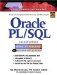Oracle PL/SQL Interactive Workbook (2nd Edition)
ISBN: 0130473200
EAN: 2147483647
Year: 2002
Pages: 146